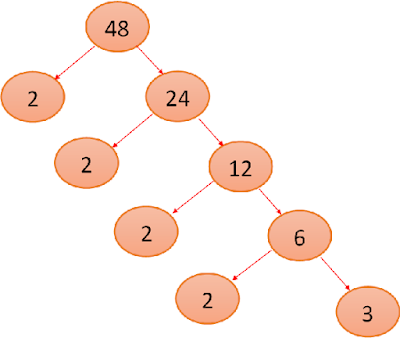Factors of 48 are the integers which will provide the original number i.e. 48 when multiplies together in pairs and also factors of 48 are the numbers that divide 48 completely that means with 0 remainders. It can be in a single factor and in factors pairs. Finding the factor of 48 is a simple method and the same procedure is followed to calculate other numbers’ factors.

 Factors of 48 Factor Pairs of 48 1, 2, 3, 4, 6, 8, 12, 16, 24 and 48 1 x 48 2 x 24 3 x 16 4 x 12 6 x 8

The total no of factors of 48 is 10.

Here we did not consider the negative integers as factors. But they are also the factors of 48.

If we multiply -2 to -24 then we also get 48.
Negative factors of 48 are:

 Factors of 48 Factor Pairs of 48 -1, -2, -3, -4, -6, -8, -12, -16, -24 and -48 -1 x -48 -2 x -24 -3 x -16 -4 x -12 -6 x -8

So the positive integer factors are also the negative factors

Now the total no of factors of 48 are: 20
-48, -24, -16, -12, -8, -6, -4, -3, -2, -1, 1, 2, 3, 4, 6, 8, 12, 16, 24, 84

Here some divisible examples of the factors of 48:

These are the example of some factors and the same for other factors of 48

## Prime Factors of 48:

 Prime Factors of 48 Prime Factorization of 48 2 x 2 x 2 x 2 x 3 or 24 x 3 48 ÷ 2 = 24 24 ÷ 2 = 12 12 ÷ 2 = 6 6 ÷ 2 = 3 3 ÷ 3 = 1

Prime factor means to factorize or break the number into the smallest divisible numbers.

Prime numbers can only be divided by itself or by 1. 48 is a composite number and it is factorized till we get all prime no of its composites.

Here we get the smallest no 2 and 3 which cannot further be divided or factorized. So these are the prime factors of 48.
We can calculate the prime factors of 48 by creating a tree and also called a factor tree.

A factor tree is a multi-step diagram that helps you to track the multiple factoring steps.Here also again we get the same prime factors of 48 that are:
2 x 2 x 2 x 2 x 3

Mean of factors of 48: 12.4

Median is 8

Multiples of 48: 48, 96, 144, 192, 240, 288, 336, 384, 432, 480, 528, 576, 624 ……… so on.

## Important Point to be remembered:

Factorization is the process to break the product into its factors from which this product is made.

Factors are any two numbers that can multiply together to get any 3rd number.

Remember that the factors always includes 1 and itself.
A factor is just a number that can be divided into whatever number without referring the remainder.

A factor is another way of looking at that is seeing that any two numbers that can multiply together and give you that other number.

Prime factors are the smallest no that can’t be further divided or factorize into smaller numbers or they are called prime numbers.
Examples of prime no are 2, 3, 5, 7, 11, 13, 17, 23 …… so on.
They can only be divided by itself or by 1.

Below are the some question that are asked many times:

### 48 is a composite number or not?

Yes, 48 is a composite number. It contains factors other than itself and 1. That means it and divided evenly into other numbers.

### What is the Prime factorization of 48?

2 x 2 x 2 x 2 x 3

### What are the factors of 48?

1, 2, 3, 4, 6, 8, 12, 16, 24, 48

### Is 48 a square number?

No, 48 is not a square no. its sq. root it 6.93.

124

### What is the highest factor of 48?

48 is itself its highest factor but if we talk about its greatest common factor i.e. GCF that is 8.

### What are the multiples of 48?

48, 96, 144, 192, 240, 288, 336, 384, 432, 480, 528, 576, 624 ……… so on.